Updated: 8/20/2022

# Measures of Association

0%
Topic
Review Topic
0
0
N/A
N/A
Questions
5
0
0
0%
0%
Evidence
3
0
0
0%
0%
Videos / Pods
1
Topic
Images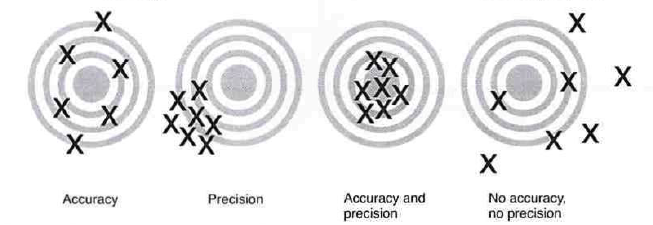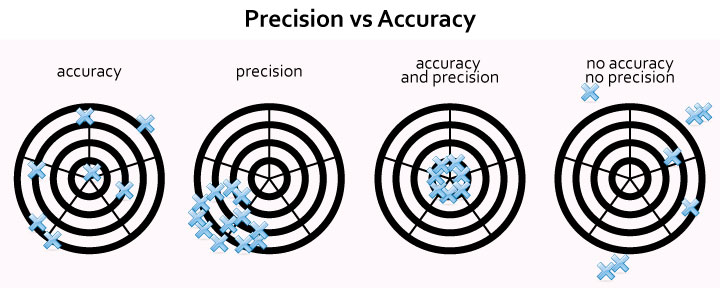• Overview
• Measures of association quantify relationships between variables
• In medicine and public health, measures of association most often quantify the association of exposure with disease
• Measures of association can be presented and framed in different ways for diverse purposes
• 2x2 tables can be helpful in calculating these measures
• note: these tables are slightly different from 2x2 tables used to calculate diagnostic testing parameters
•  Relative Risk, Odds Ratio,Attributable Risk, and Absolute Risk Reduction Relative risk (RR)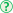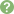risk of disease in exposed group/risk of disease in unexposed group= [a/(a+b)] / [c/(c+d)]used in cohort studies or other studies where total population is knownRR > 1exposure is associated with increased risk of diseaseRR < 1exposure is associated with decreased risk of diseaseRR = 1no association between exposure and diseaseOdds ratio (OR)odds of having disease in exposed group/odds of having disease in unexposed group= ad/bcprimarily used in case control studiescan be used in cohort studies when outcome is rareOR approximates RR for rare outcomesOR > 1odds of developing disease are greater in exposed groupOR < 1odds of developing disease are reduced in exposed groupOR = 1odds of developing disease are equal in exposed and unexposed groupAttributable risk (AR)"How much greater risk is present in the exposed group than the unexposed?"risk of disease in exposed group - risk in unexposed group= a/(a+b) - c/(c+d)Attributable risk percent (ARP)"What percent greater risk is present in the exposed group than the unexposed?"(risk of disease in exposed group - risk in unexposed group)/risk in exposed group= [a/(a+b) - c/(c+d)] / [a/(a+b)]Absolute risk reduction (ARR)"How much risk is reduced by the intervention (or exposure)?"risk in control group - risk in intervention group= c/(c+d) - a/(a+b) Number Needed to Treat and Number Needed to Harm Number needed to treat (NNT)"How many patients needed to be treated before 1 patient will benefit?"e.g.,if a treatment affords a 25% ARR, generally 4 people will have to be treated for every 1 that benefitsNNT calculation is the inverse of ARR=1/[c/(c+d) - a/(a+b)]Number needed to harm (NNH)"How many patients need to be exposed before one patient will be harmed?"NNH calculation is the inverse of AR= 1/[a/(a+b) - c/(c+d)] Population-Level Measures of Association Used to make public health decisions and allocate resourcesPopulation attributable risk (PAR)"What amount of the risk of disease in a population is attributable to a specific exposure?" (as opposed to baseline population risk)total population incidence of disease - incidence of disease amongst unexposed= [(a+c)/(a+b+c+d) - (c/c+d)]unit is per persone.g., if your PAR for obesity on heart disease is 0.004, the risk of heart disease for the total population that is likely due to obesity is 4 cases per 1,000 peopleif obesity was eliminated from the population, 4 cases of cardiac disease per every 1,000 people would be eliminatedPopulation attributable risk percent (PAR%)"What percent of disease cases amongst a population can be attributed to a specific exposure?" (as opposed to baseline population risk)[(total population incidence of disease - incidence of disease amongst unexposed)/total population incidence of disease] * 100= [(a+c)/(a+b+c+d) - (c/c+d)]/[(a+c)/(a+b+c+d)] * 100

You have never rated this topic.

Thank you. You can rate this topic again in 12 months.

Flashcards (0)
Cards
1 of 0
Questions (5)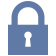Sorry, this question is forSorry, this question is for

(M1.ST.14.14) A group of neurologists develop a new blood test for Alzheimer's. They are optimistic about the test, as they have found that for any given patient, the test repeatedly produces very similar results. However, they find that the new test results are not necessarily consistent with the gold standard of diagnosis. How would this new test most accurately be described?

QID: 103976

Valid

1%

(1/78)

Reliable

68%

(53/78)

Biased

9%

(7/78)

Valid and reliable

4%

(3/78)

Neither valid nor reliable

12%

(9/78)

M 1 D

Select Answer to see Preferred ResponseSorry, this question is for